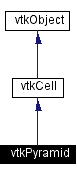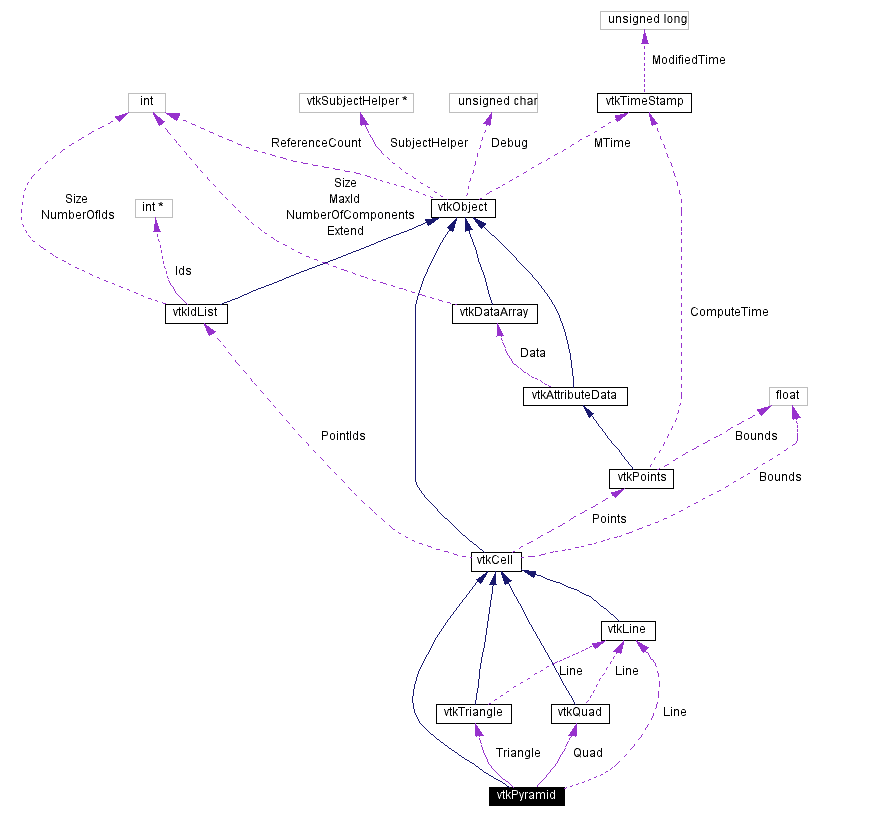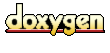Main Page   Class Hierarchy   Alphabetical List   Compound List   File List   Compound Members   File Members   Related Pages

# vtkPyramid Class Reference

a 3D cell that represents a pyramid. More...

`#include <vtkPyramid.h>`

Inheritance diagram for vtkPyramid:[legend]
Collaboration diagram for vtkPyramid:[legend]
List of all members.

## Public Methods

virtual const char * GetClassName ()
virtual int IsA (const char *type)
vtkCellMakeObject ()
int GetCellType ()
int GetCellDimension ()
int GetNumberOfEdges ()
int GetNumberOfFaces ()
vtkCellGetEdge (int edgeId)
vtkCellGetFace (int faceId)
int CellBoundary (int subId, float pcoords, vtkIdList *pts)
void Contour (float value, vtkScalars *cellScalars, vtkPointLocator *locator, vtkCellArray *verts, vtkCellArray *lines, vtkCellArray *polys, vtkPointData *inPd, vtkPointData *outPd, vtkCellData *inCd, int cellId, vtkCellData *outCd)
void Clip (float value, vtkScalars *cellScalars, vtkPointLocator *locator, vtkCellArray *cells, vtkPointData *inPd, vtkPointData *outPd, vtkCellData *inCd, int cellId, vtkCellData *outCd, int insideOut)
int EvaluatePosition (float x, float *closestPoint, int &subId, float pcoords, float &dist2, float *weights)
void EvaluateLocation (int &subId, float pcoords, float x, float *weights)
int IntersectWithLine (float p1, float p2, float tol, float &t, float x, float pcoords, int &subId)
int Triangulate (int index, vtkIdList *ptIds, vtkPoints *pts)
void Derivatives (int subId, float pcoords, float *values, int dim, float *derivs)
int GetParametricCenter (float pcoords)
int JacobianInverse (float pcoords, double **inverse, float derivs)

## Static Public Methods

int IsTypeOf (const char *type)
vtkPyramid * SafeDownCast (vtkObject *o)
vtkPyramid * New ()
int * GetFaceArray (int faceId)
void InterpolationFunctions (float pcoords, float weights)
void InterpolationDerivs (float pcoords, float derivs)

## Protected Methods

vtkPyramid ()
~vtkPyramid ()
vtkPyramid (const vtkPyramid &)
void operator= (const vtkPyramid &)

## Protected Attributes

vtkLineLine
vtkTriangleTriangle

## Detailed Description

a 3D cell that represents a pyramid.

Date:
2000/12/10 20:08:16
Revision:
1.10

vtkPyramid is a concrete implementation of vtkCell to represent a 3D pyramid. A pyramid consists of a rectangular base with four triangular faces.

Examples:
vtkPyramid (examples)

Definition at line 64 of file vtkPyramid.h.

## Constructor & Destructor Documentation

 vtkPyramid::vtkPyramid ( ) ` [protected]`

 vtkPyramid::~vtkPyramid ( ) ` [protected]`

 vtkPyramid::vtkPyramid ( const vtkPyramid & ) ` [inline, protected]`
 Definition at line 114 of file vtkPyramid.h.

## Member Function Documentation

 virtual const char* vtkPyramid::GetClassName ( ) ` [virtual]`
 Return the class name as a string. This method is defined in all subclasses of vtkObject with the vtkTypeMacro found in vtkSetGet.h. Reimplemented from vtkCell.

 int vtkPyramid::IsTypeOf ( const char * type ) ` [static]`
 Return 1 if this class type is the same type of (or a subclass of) the named class. Returns 0 otherwise. This method works in combination with vtkTypeMacro found in vtkSetGet.h. Reimplemented from vtkCell.

 virtual int vtkPyramid::IsA ( const char * type ) ` [virtual]`
 Return 1 if this class is the same type of (or a subclass of) the named class. Returns 0 otherwise. This method works in combination with vtkTypeMacro found in vtkSetGet.h. Reimplemented from vtkCell.

 vtkPyramid* vtkPyramid::SafeDownCast ( vtkObject * o ) ` [static]`
 Will cast the supplied object to vtkObject* is this is a safe operation (i.e., a safe downcast); otherwise NULL is returned. This method is defined in all subclasses of vtkObject with the vtkTypeMacro found in vtkSetGet.h. Reimplemented from vtkCell.

 vtkPyramid* vtkPyramid::New ( ) ` [static]`
 Create an instance of this class. Reimplemented from vtkObject.

 vtkCell* vtkPyramid::MakeObject ( ) ` [virtual]`
 See the vtkCell API for descriptions of these methods. Reimplemented from vtkCell.

 int vtkPyramid::GetCellType ( ) ` [inline, virtual]`
 Return the type of cell. Reimplemented from vtkCell. Definition at line 74 of file vtkPyramid.h.

 int vtkPyramid::GetCellDimension ( ) ` [inline, virtual]`
 Return the topological dimensional of the cell (0,1,2, or 3). Reimplemented from vtkCell. Definition at line 75 of file vtkPyramid.h.

 int vtkPyramid::GetNumberOfEdges ( ) ` [inline, virtual]`
 Return the number of edges in the cell. Reimplemented from vtkCell. Definition at line 76 of file vtkPyramid.h.

 int vtkPyramid::GetNumberOfFaces ( ) ` [inline, virtual]`
 Return the number of faces in the cell. Reimplemented from vtkCell. Definition at line 77 of file vtkPyramid.h.

 vtkCell* vtkPyramid::GetEdge ( int edgeId ) ` [virtual]`
 Return the edge cell from the edgeId of the cell. Reimplemented from vtkCell.

 vtkCell* vtkPyramid::GetFace ( int faceId ) ` [virtual]`
 Return the face cell from the faceId of the cell. Reimplemented from vtkCell.

 int* vtkPyramid::GetFaceArray ( int faceId ) ` [static]`

 int vtkPyramid::CellBoundary ( int subId, float pcoords, vtkIdList * pts ) ` [virtual]`
 Given parametric coordinates of a point, return the closest cell boundary, and whether the point is inside or outside of the cell. The cell boundary is defined by a list of points (pts) that specify a face (3D cell), edge (2D cell), or vertex (1D cell). If the return value of the method is != 0, then the point is inside the cell. Reimplemented from vtkCell.

 void vtkPyramid::Contour ( float value, vtkScalars * cellScalars, vtkPointLocator * locator, vtkCellArray * verts, vtkCellArray * lines, vtkCellArray * polys, vtkPointData * inPd, vtkPointData * outPd, vtkCellData * inCd, int cellId, vtkCellData * outCd ) ` [virtual]`
 Generate contouring primitives. The scalar list cellScalars are scalar values at each cell point. The point locator is essentially a points list that merges points as they are inserted (i.e., prevents duplicates). Contouring primitives can be vertices, lines, or polygons. It is possible to interpolate point data along the edge by providing input and output point data - if outPd is NULL, then no interpolation is performed. Also, if the output cell data is non-NULL, the cell data from the contoured cell is passed to the generated contouring primitives. (Note: the CopyAllocate() method must be invoked on both the output cell and point data. The cellId refers to the cell from which the cell data is copied.) Reimplemented from vtkCell.

 void vtkPyramid::Clip ( float value, vtkScalars * cellScalars, vtkPointLocator * locator, vtkCellArray * cells, vtkPointData * inPd, vtkPointData * outPd, vtkCellData * inCd, int cellId, vtkCellData * outCd, int insideOut ) ` [virtual]`
 Cut (or clip) the cell based on the input cellScalars and the specified value. The output of the clip operation will be one or more cells of the same topological dimension as the original cell. The flag insideOut controls what part of the cell is considered inside - normally cell points whose scalar value is greater than "value" are considered inside. If insideOut is on, this is reversed. Also, if the output cell data is non-NULL, the cell data from the clipped cell is passed to the generated contouring primitives. (Note: the CopyAllocate() method must be invoked on both the output cell and point data. The cellId refers to the cell from which the cell data is copied.) Reimplemented from vtkCell.

 int vtkPyramid::EvaluatePosition ( float x, float * closestPoint, int & subId, float pcoords, float & dist2, float * weights ) ` [virtual]`
 Given a point x return inside(=1) or outside(=0) cell; evaluate parametric coordinates, sub-cell id (!=0 only if cell is composite), distance squared of point x to cell (in particular, the sub-cell indicated), closest point on cell to x (unless closestPoint is null, in which case, the closest point and dist2 are not found), and interpolation weights in cell. (The number of weights is equal to the number of points defining the cell). Note: on rare occasions a -1 is returned from the method. This means that numerical error has occurred and all data returned from this method should be ignored. Also, inside/outside is determine parametrically. That is, a point is inside if it satisfies parametric limits. This can cause problems for cells of topological dimension 2 or less, since a point in 3D can project onto the cell within parametric limits but be "far" from the cell. Thus the value dist2 may be checked to determine true in/out. Reimplemented from vtkCell.

 void vtkPyramid::EvaluateLocation ( int & subId, float pcoords, float x, float * weights ) ` [virtual]`
 Determine global coordinate (x) from subId and parametric coordinates. Also returns interpolation weights. (The number of weights is equal to the number of points in the cell.) Reimplemented from vtkCell.

 int vtkPyramid::IntersectWithLine ( float p1, float p2, float tol, float & t, float x, float pcoords, int & subId ) ` [virtual]`
 Intersect with a ray. Return parametric coordinates (both line and cell) and global intersection coordinates, given ray definition and tolerance. The method returns non-zero value if intersection occurs. Reimplemented from vtkCell.

 int vtkPyramid::Triangulate ( int index, vtkIdList * ptIds, vtkPoints * pts ) ` [virtual]`
 Generate simplices of proper dimension. If cell is 3D, tetrahedron are generated; if 2D triangles; if 1D lines; if 0D points. The form of the output is a sequence of points, each n+1 points (where n is topological cell dimension) defining a simplex. The index is a parameter that controls which triangulation to use (if more than one is possible). If numerical degeneracy encountered, 0 is returned, otherwise 1 is returned. Reimplemented from vtkCell.

 void vtkPyramid::Derivatives ( int subId, float pcoords, float * values, int dim, float * derivs ) ` [virtual]`
 Compute derivatives given cell subId and parametric coordinates. The values array is a series of data value(s) at the cell points. There is a one-to-one correspondence between cell point and data value(s). Dim is the number of data values per cell point. Derivs are derivatives in the x-y-z coordinate directions for each data value. Thus, if computing derivatives for a scalar function in a hexahedron, dim=1, 8 values are supplied, and 3 deriv values are returned (i.e., derivatives in x-y-z directions). On the other hand, if computing derivatives of velocity (vx,vy,vz) dim=3, 24 values are supplied ((vx,vy,vz)1, (vx,vy,vz)2, ....()8), and 9 deriv values are returned ((d(vx)/dx),(d(vx)/dy),(d(vx)/dz), (d(vy)/dx),(d(vy)/dy), (d(vy)/dz), (d(vz)/dx),(d(vz)/dy),(d(vz)/dz)). Reimplemented from vtkCell.

 int vtkPyramid::GetParametricCenter ( float pcoords ) ` [inline, virtual]`
 Return the center of the pyramid in parametric coordinates. Reimplemented from vtkCell. Definition at line 123 of file vtkPyramid.h.

 void vtkPyramid::InterpolationFunctions ( float pcoords, float weights ) ` [static]`
 Pyramid specific methods for computing interpolation functions and derivatives.

 void vtkPyramid::InterpolationDerivs ( float pcoords, float derivs ) ` [static]`

 int vtkPyramid::JacobianInverse ( float pcoords, double ** inverse, float derivs )

 void vtkPyramid::operator= ( const vtkPyramid & ) ` [inline, protected]`
 Definition at line 115 of file vtkPyramid.h.

## Member Data Documentation

 vtkLine* vtkPyramid::Line` [protected]`
 Definition at line 117 of file vtkPyramid.h.

 vtkTriangle* vtkPyramid::Triangle` [protected]`
 Definition at line 118 of file vtkPyramid.h.

 vtkQuad* vtkPyramid::Quad` [protected]`
 Definition at line 119 of file vtkPyramid.h.

The documentation for this class was generated from the following file:

Generated on Wed Nov 21 12:58:14 2001 for VTK by1.2.11.1 written by Dimitri van Heesch, © 1997-2001